## 混沌

• 蝴蝶效应(初始值的微小变化极大影响结果)；
• 非线性现象(多个因素相互影响/约束，未知函数有非线性项)，其实就是蝴蝶效应的原因；
• 典型的混沌现象
• 天气预报，长期天气预报注定失败(微小的变化会影响很远很远)。
• 三体问题。
• 混沌和随机运动的差异：外在表现和纯粹的随机运动很相似，即都不可预测。但和随机运动不同的是，混沌运动在动力学上是确定的，它的不可预测性是来源于运动的不稳定性，或者说混沌系统对无限小的初值变动和微扰也具有敏感性，无论多小的扰动在长时间以后，也会使系统彻底偏离原来的演化方向。

(这句话是说必然性、确定性的思维方式，而混沌与分形在思想方法上对我们来说是一个冲击。)

To see a World in a Grain of Sand And a Heaven in a Wild Flower, Hold Infinity in the palm of your hand And Eternity in an hour. — William Blake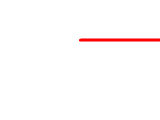洛伦兹吸引子，以其双纽线形状而著称，映射展示出动力系统(三维系统的三个变量)的状态是如何以一种复杂且不重复的模式，随时间的推移而演变的，对应方程组(第二个和第三个方程非线性)如下。(交互动画参考Lorenz System—geogebra)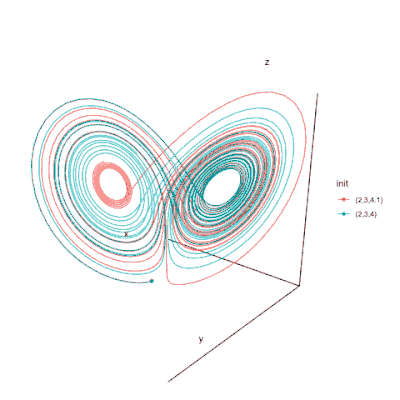\begin{aligned} &\frac{d x}{d t}=10(x-y) \\ &\frac{d y}{d t}=28 x-y-x z \\ &\frac{d z}{d t}=x y-\frac{8}{3} z \end{aligned}

• 其中$$x$$、$$y$$和$$z$$分别表示对流强弱、上升部分和下降部分的温差、水平方向的温差；
• 上图表示的其实是一个相空间；
• 吸引子是动态系统的归宿。归宿，比如往天上扔个东西，或者树上的苹果，最后都会落到地上，这就是归宿。对于洛伦兹吸引子来说，归宿就是蝴蝶；对于地球围绕太阳转，由于没有摩擦力，只有万有引力，系统没有耗散，所以吸引子是一个极限环。
• 分形维数大约2.06；
• 分形是混沌在空间中的描述，混沌是分形在时间上的体现。混沌现象可以看作由连续空间(时域)向分形流形(频域/空域)的映射。

Logistic映射(驱动力耗散力)，这个例子类似“种群”的增长/衰退，$$x_{n}$$表示种群在第$$n$$代时的数量：$$x_{n+1}=f\left(x_{n}\right)=\mu x_{n}\left(1-x_{n}\right)$$存在定常解$$x_{*}=0$$和$$x_{*}=1-\displaystyle\frac{1}{\mu}$$。

(1) 如果有$$a$$使得$$f(a)=a$$，则称$$a$$为不动点
(2) 如果有$$a$$使得$$f^{k}(a)=a$$，则称$$a$$为周期点;
(3) 使得上式成立的最小的$$k$$，称为周期

1. 《蝴蝶效应之谜：走近分形与混沌》(张天蓉)
2. 书的读书笔记-CSDN
3. 从刘徽割圆谈起
4. 妈咪说MommyTalk视频-混沌与分形专题
5. 雪花周长和地球直径谁更大？1.26维空间啥样？李永乐老师讲分形几何

## 分形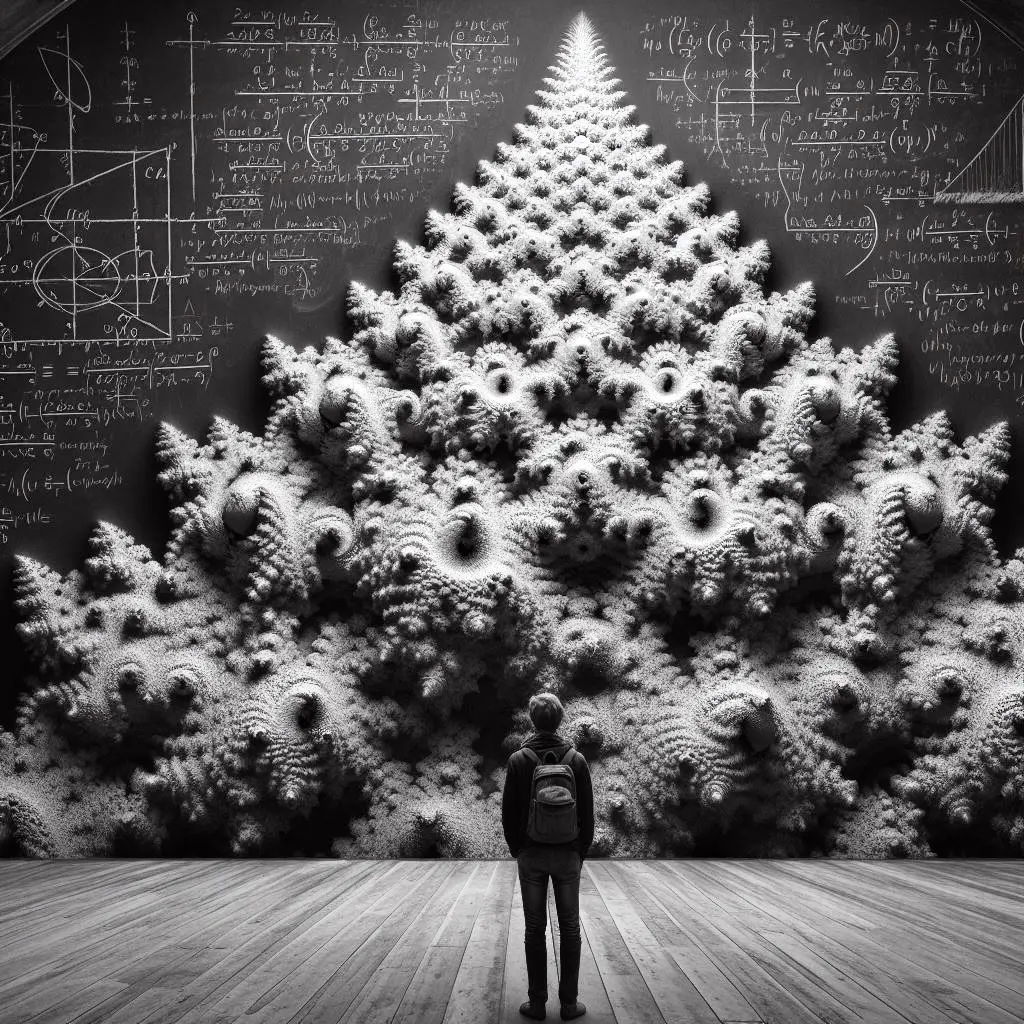A fractal is a way of seeing infinity.——Benoit Mandelbrot

1967年，英国工程师在科学杂志上发表文章《英国的海岸线有多长》，他提到从一定的意义上来说，英国的海岸线是无限长，量的尺子越小，最终的结果是海岸线测量结果越长。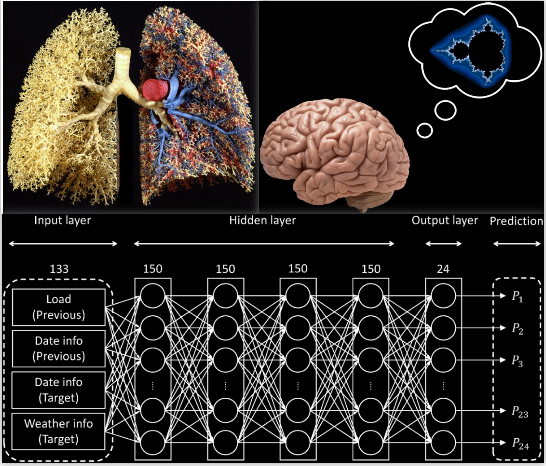Some of the most visually striking examples of fractal forms are found in physiology: The respiratory, circulatory, and nervous systems are remarkable instances of fractal architecture, branches subdividing and subdividing and subdividing again. 上图画了肺的分形结构。大脑不是分形的，但是mind可以产生分形结构的思考过程；从人工智能和机器学习的角度看，some of the most successful of these deep neural networks operate on a principle where they're processing information over and over again, while periodically feeding back in the original information to keep the process going. And that might sound a little bit familiar, because it actually is quite similar to the way that Mandelbrot's fractal equation works. It's an engine for generating fractal levels of complexity. And perhaps that's why these fractal images resonate so deeply with us. It's because when we're looking at them, in a way, we're seeing a reflection of ourselves.

• 利希滕贝格图；
• 树木、蕨类植物、菜花；
• 身体中的血管，神经；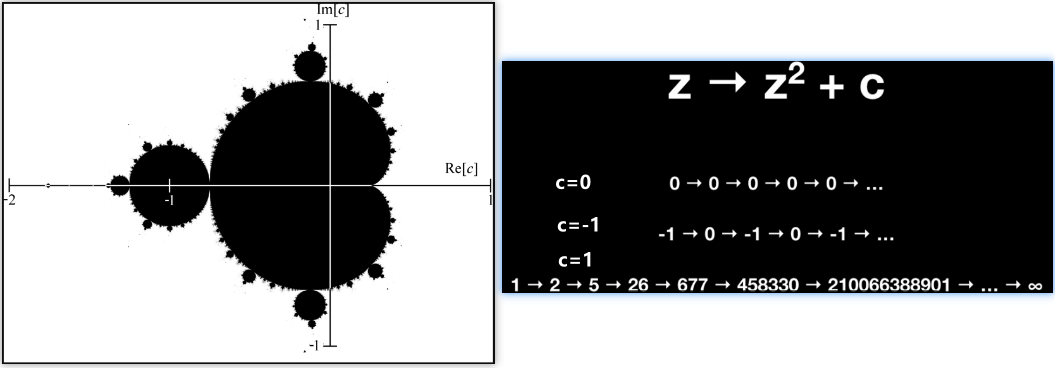(1) 任意小的尺度上都具有精细结构；
(2) 无法用传统的欧式几何来描述；
(3) 具有自相似性(self-similarity)，其实就是套娃；
(4) 具有简单的递归定义；
(5) 一般情况下分形维数会大于拓扑维数，且可以是分数(皮亚诺曲线和希尔伯特曲线就是例外)；

• 传统维数：我们传统的线是一维，面是二维，体是三维，这些是拓扑维度，从这个角度讲上面画的科赫雪花和谢尔宾斯基地毯都是二维的；
• 豪斯多夫维数是专门用来研究分形结构的；
• 皮亚诺曲线和希尔伯特曲线的分形维数是2；
• 科赫雪花维数的计算：将科赫曲线的尺寸缩小至原来的三分之一；然后，用4个这样的小科赫曲线，便能构成与原来一模一样的科赫曲线。因此，我们得到科赫曲线的维数为$$d=\frac{\ln 4}{\ln 3}=1.2618 \cdots$$。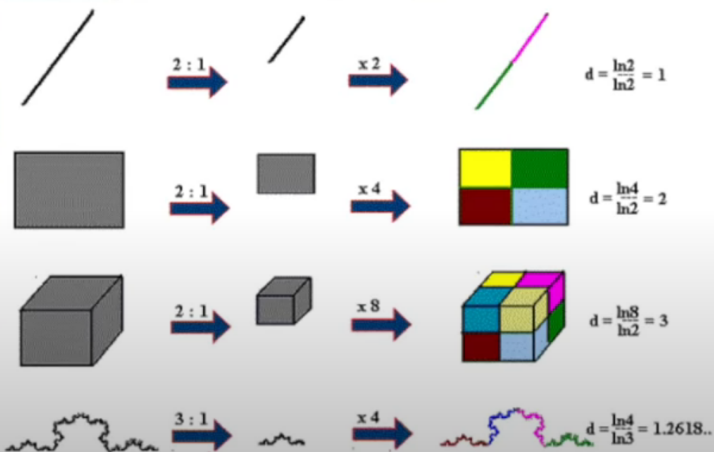Hausdorff测度：

Lebesgue测度：设$$E$$是$$\mathbf{R}^{n}$$上的一点集，设$$\left\{U_{k}\right\}$$是$$E$$的开覆盖，定义直径：$$diaU=\sup \{d(x, y) \mid \forall x, y \in U\}$$定义$$\inf \displaystyle\sum_{k}\left(diaU_{k}\right)^{n}$$为集合$$E$$的Lebesgue外测度。(注：可能相差一个正常数)

(1) Fractals: a world in a grain of sand | Ben Weiss | TEDxVeniceBeach
(2) Fractals in Physiology—Yale Math
(3) Are Humans Fractals? Biology and Behavior (multiple studies)—Conscious Vibe
(4) 《蝴蝶效应之谜：走近分形与混沌》— 张天蓉
(5) 《大自然的分形几何学》—Mandelbrot